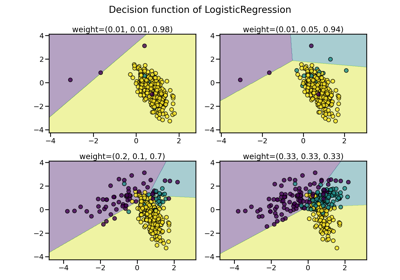# imblearn.over_sampling.SMOTENC¶

class imblearn.over_sampling.SMOTENC(categorical_features, *, sampling_strategy='auto', random_state=None, k_neighbors=5, n_jobs=None)[source]

Synthetic Minority Over-sampling Technique for Nominal and Continuous.

Unlike SMOTE, SMOTE-NC for dataset containing continuous and categorical features. However, it is not designed to work with only categorical features.

Read more in the User Guide.

Parameters
categorical_featuresndarray of shape (n_cat_features,) or (n_features,)

Specified which features are categorical. Can either be:

• array of indices specifying the categorical features;

• mask array of shape (n_features, ) and bool dtype for which True indicates the categorical features.

sampling_strategyfloat, str, dict or callable, default=’auto’

Sampling information to resample the data set.

• When float, it corresponds to the desired ratio of the number of samples in the minority class over the number of samples in the majority class after resampling. Therefore, the ratio is expressed aswhereis the number of samples in the minority class after resampling andis the number of samples in the majority class.

Warning

float is only available for binary classification. An error is raised for multi-class classification.

• When str, specify the class targeted by the resampling. The number of samples in the different classes will be equalized. Possible choices are:

'minority': resample only the minority class;

'not minority': resample all classes but the minority class;

'not majority': resample all classes but the majority class;

'all': resample all classes;

'auto': equivalent to 'not majority'.

• When dict, the keys correspond to the targeted classes. The values correspond to the desired number of samples for each targeted class.

• When callable, function taking y and returns a dict. The keys correspond to the targeted classes. The values correspond to the desired number of samples for each class.

random_stateint, RandomState instance, default=None

Control the randomization of the algorithm.

• If int, random_state is the seed used by the random number generator;

• If RandomState instance, random_state is the random number generator;

• If None, the random number generator is the RandomState instance used by np.random.

k_neighborsint or object, default=5

If int, number of nearest neighbours to used to construct synthetic samples. If object, an estimator that inherits from sklearn.neighbors.base.KNeighborsMixin that will be used to find the k_neighbors.

n_jobsint, default=None

Number of CPU cores used during the cross-validation loop. None means 1 unless in a joblib.parallel_backend context. -1 means using all processors. See Glossary for more details.

SMOTE

Over-sample using SMOTE.

SVMSMOTE

Over-sample using SVM-SMOTE variant.

BorderlineSMOTE

Over-sample using Borderline-SMOTE variant.

ADASYN

KMeansSMOTE

Over-sample applying a clustering before to oversample using SMOTE.

Notes

See the original paper  for more details.

Supports mutli-class resampling. A one-vs.-rest scheme is used as originally proposed in .

References

1(1,2)

N. V. Chawla, K. W. Bowyer, L. O.Hall, W. P. Kegelmeyer, “SMOTE: synthetic minority over-sampling technique,” Journal of artificial intelligence research, 321-357, 2002.

Examples

>>> from collections import Counter
>>> from numpy.random import RandomState
>>> from sklearn.datasets import make_classification
>>> from imblearn.over_sampling import SMOTENC
>>> X, y = make_classification(n_classes=2, class_sep=2,
... weights=[0.1, 0.9], n_informative=3, n_redundant=1, flip_y=0,
... n_features=20, n_clusters_per_class=1, n_samples=1000, random_state=10)
>>> print('Original dataset shape (%s, %s)' % X.shape)
Original dataset shape (1000, 20)
>>> print('Original dataset samples per class {}'.format(Counter(y)))
Original dataset samples per class Counter({1: 900, 0: 100})
>>> # simulate the 2 last columns to be categorical features
>>> X[:, -2:] = RandomState(10).randint(0, 4, size=(1000, 2))
>>> sm = SMOTENC(random_state=42, categorical_features=[18, 19])
>>> X_res, y_res = sm.fit_resample(X, y)
>>> print('Resampled dataset samples per class {}'.format(Counter(y_res)))
Resampled dataset samples per class Counter({0: 900, 1: 900})

__init__(self, categorical_features, *, sampling_strategy='auto', random_state=None, k_neighbors=5, n_jobs=None)[source]

Initialize self. See help(type(self)) for accurate signature.

## Examples using imblearn.over_sampling.SMOTENC¶